Scroll

# Example of the script calculation feature

Follow
 Summary This document introduces calculations which use scripts; it also shows examples of how they can be used to make a table set-up more efficient and concise. Applies to Askia Analyse. Written for Data Processors, Analysts, Researchers, Statistical Analysts. Keywords Script, Base,Calculation, Statistics, Advanced options, Frequency, Mean, Standard deviation, Total, Frequency by script, Sort, Transpose, Advanced sort

Script calculations are a great way of setting up your tables in a more efficient manner. They give you the ability to achieve tables which would not be possible otherwise.

The best way to show this is to walk through an example where we remove one variable from the rows of our table set up and replace it with script calculations.

You have a table set-up in Analyse as follows: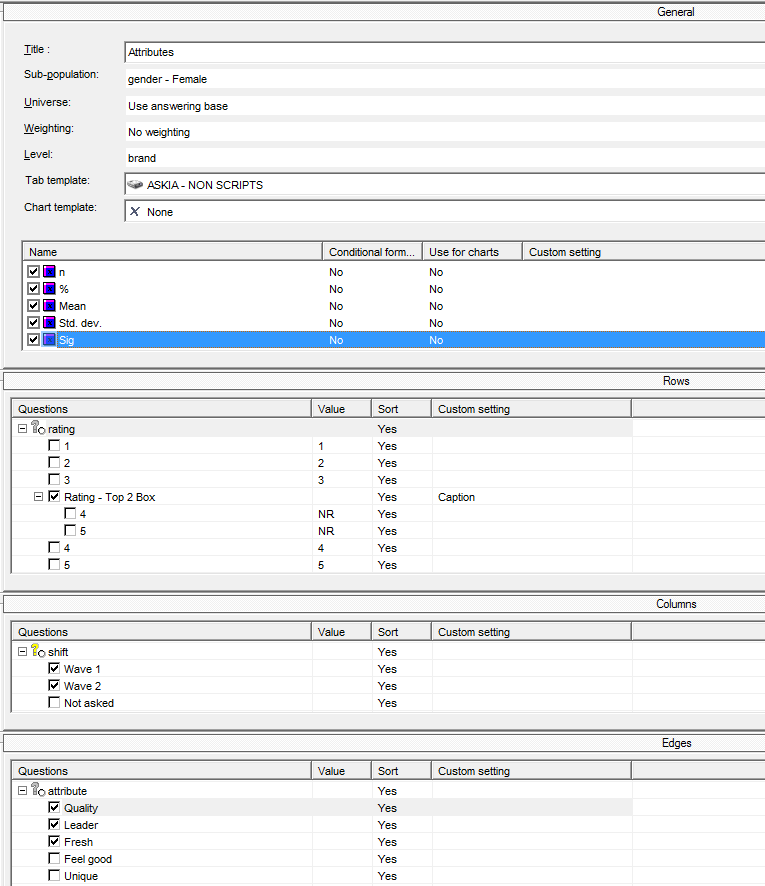You want to show the calculations n, %, Mean, Std. dev, Sig for the rows / cols / edges in the image above. However, you also need to cross these with some other variables: gender (Male/Female) and parents: (Parent / Non-Parent) and ideally have the information in one concise table.

The problem is there is no space left in the rows / cols / edges to accommodate another profile of variables. You will have to start crossing variables or running a filtered set of tabs for each category e.g. above, I have added the sub-population: gender – Female. This will be one of four or five runs I do to get my complete set: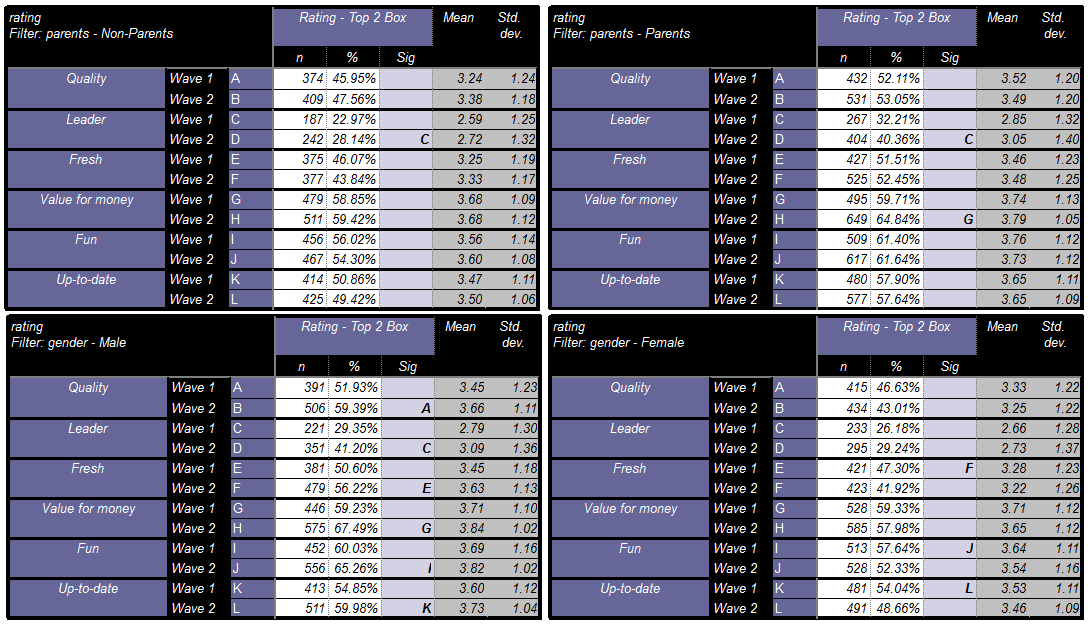It’s not ideal. So this is where script calculations can provide additional flexibility. Here is a quick overview of what will be used: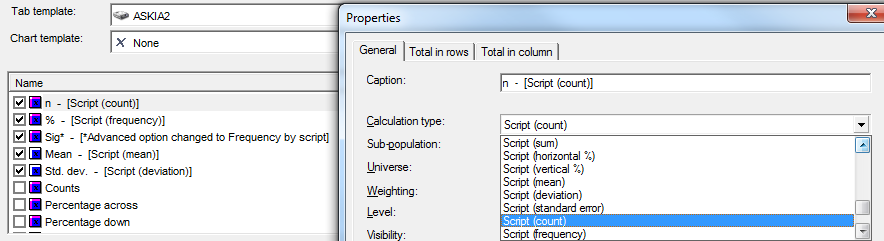The scripts used in the above calculations will all refer to ??rating?? – This variable can then be removed from the rows and we can rejig the tab definition as below. N.B. Please look at the attached .qes file and portfolio (including tab template settings) to get a better understanding of the rearrangement.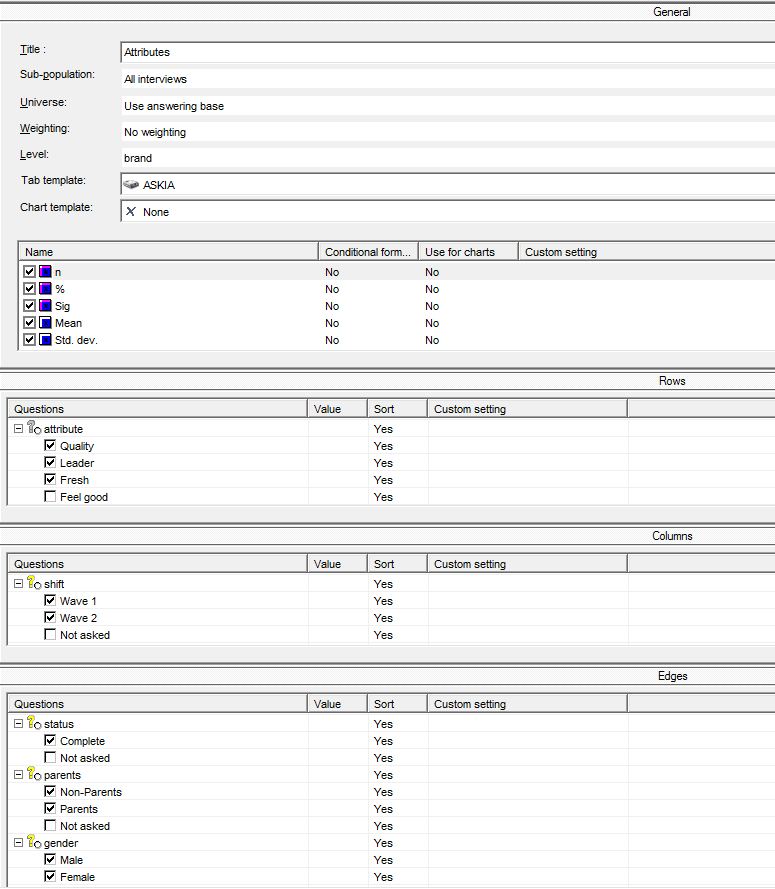We need to know what to put in the script boxes highlighted below. Each of the script calculations will require you to declare ‘Script’ but only one or two will require a ‘Base’ as well.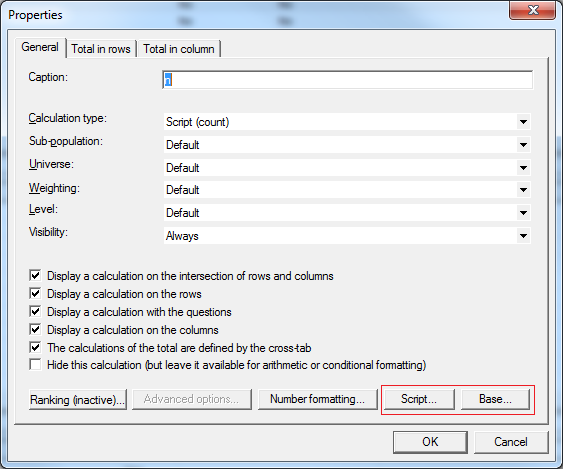n:

• [Script (count)]
• Script =  ??rating?? has {4;5}
• Base =  ??rating?? has {1 to 5}*

* We will talk further about what this ‘Base’ option does towards the end of the article

%:

• [Script (frequency)]
• Script =  ??rating?? has {4;5}
• Base =  ??rating?? has {1 to 5}

Sig:

• [Advanced option changed to Frequency by script]
• Script =  ??rating?? has {4;5}
• Base =  ??rating?? has {1 to 5}

Mean:

• [Script (mean)]
• Script =  On(??rating??,1,2,3,4,5,nr)

Std. dev:

• [Script (deviation)]
• Script =  On(??rating??,1,2,3,4,5,nr)

It is important to note here that when using scripts, you will need to adjust your sig testing advanced options to work with these script calculations rather than the regular counts and % i.e. change from Frequency to Frequency by script and then define them: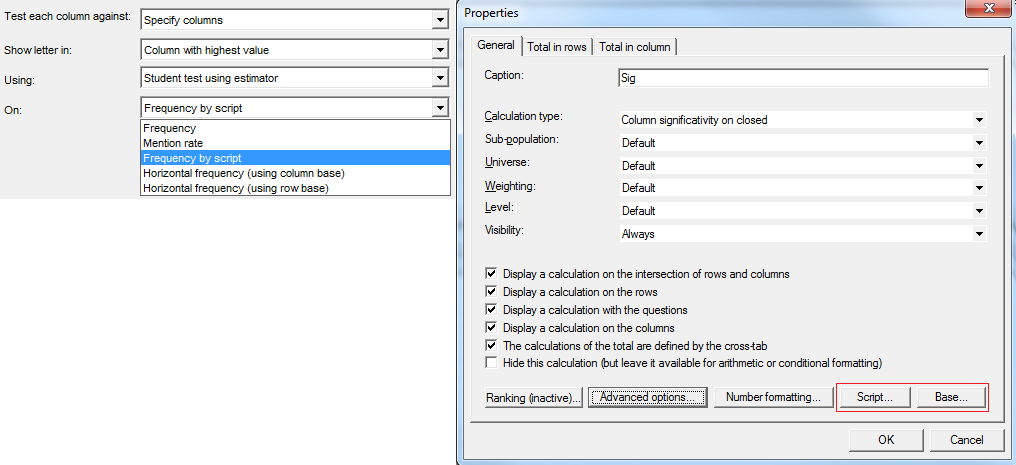The end result is a more concise Top 2 Box table like below: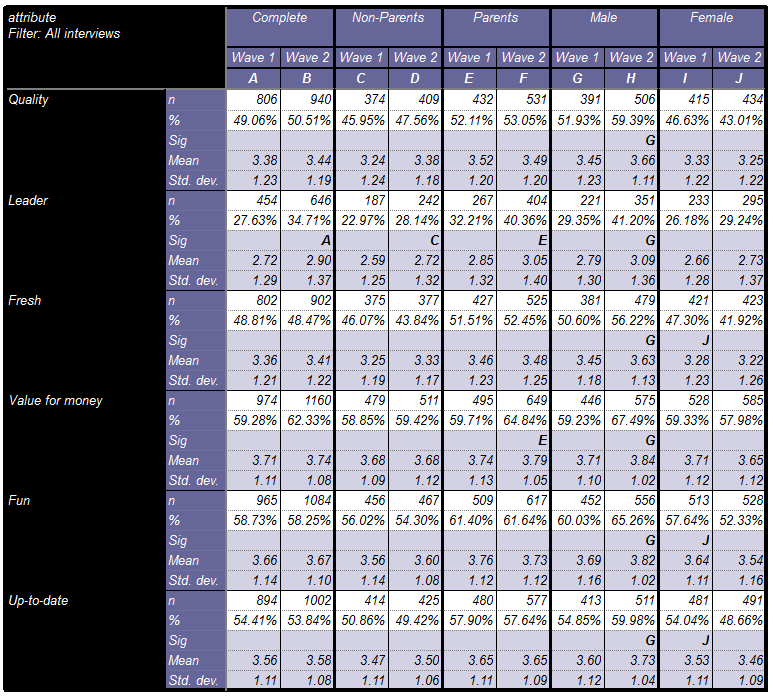The other advantage is that you can add a total column to put beside your parent and gender columns and then you can sort on any of these columns.

For example, to sort on ‘Complete’ you will right click in the rows and select advanced sort. There you will select the variable which contains this complete code i.e. ‘status’ and select its code ‘complete’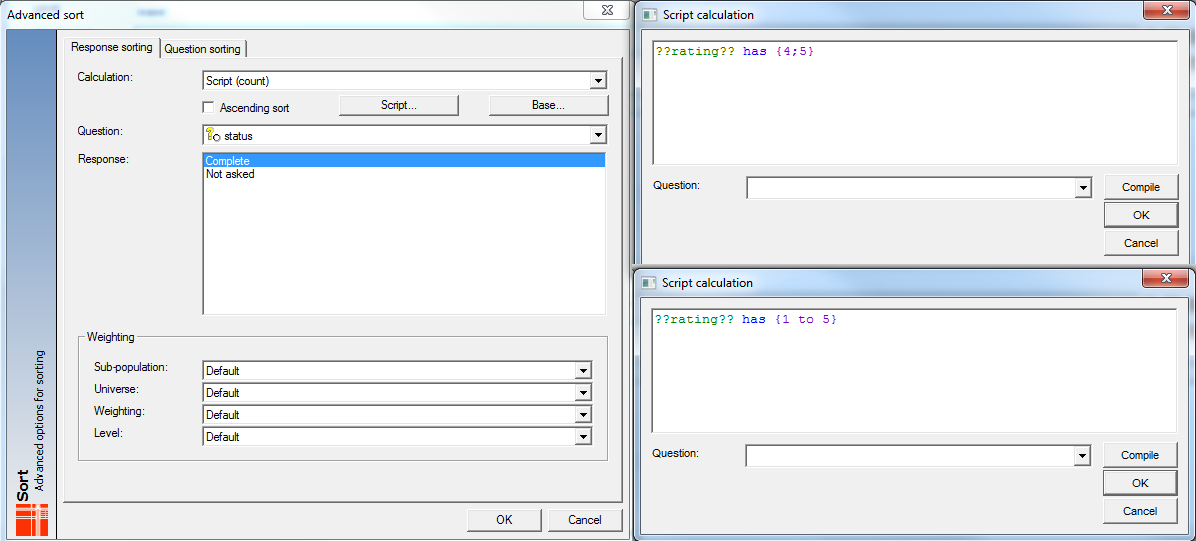This sorts on the total of complete across waves 1 & 2.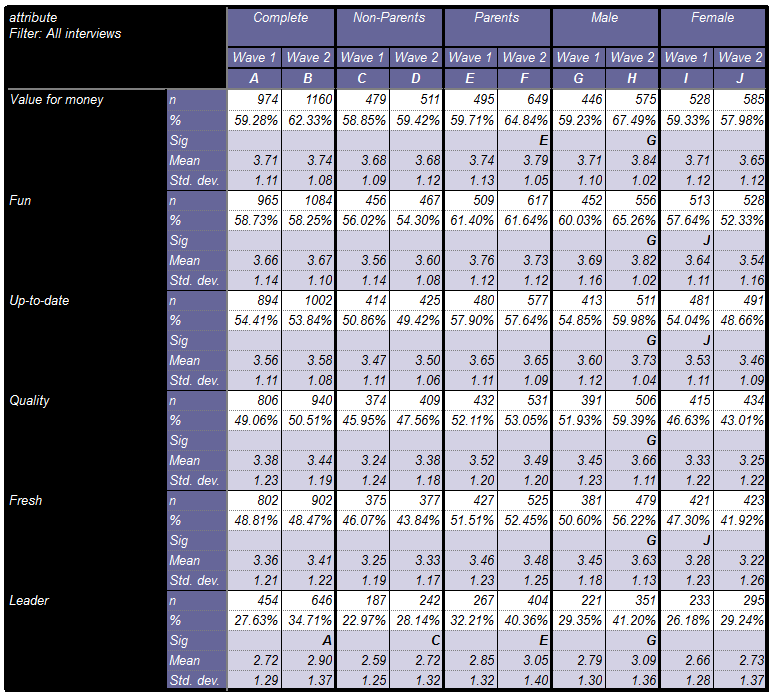If you wanted to sort on, say, Complete (or any other code) within a particular wave, you need to create a variable with that code which is also filtered by the wave you are interested. So let’s create the code for Non-parents by Wave 1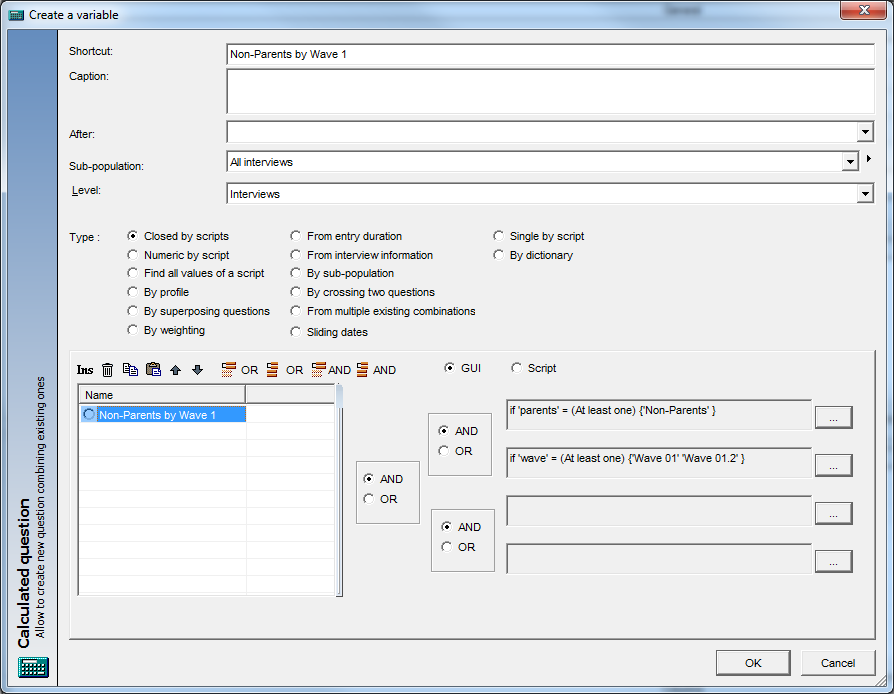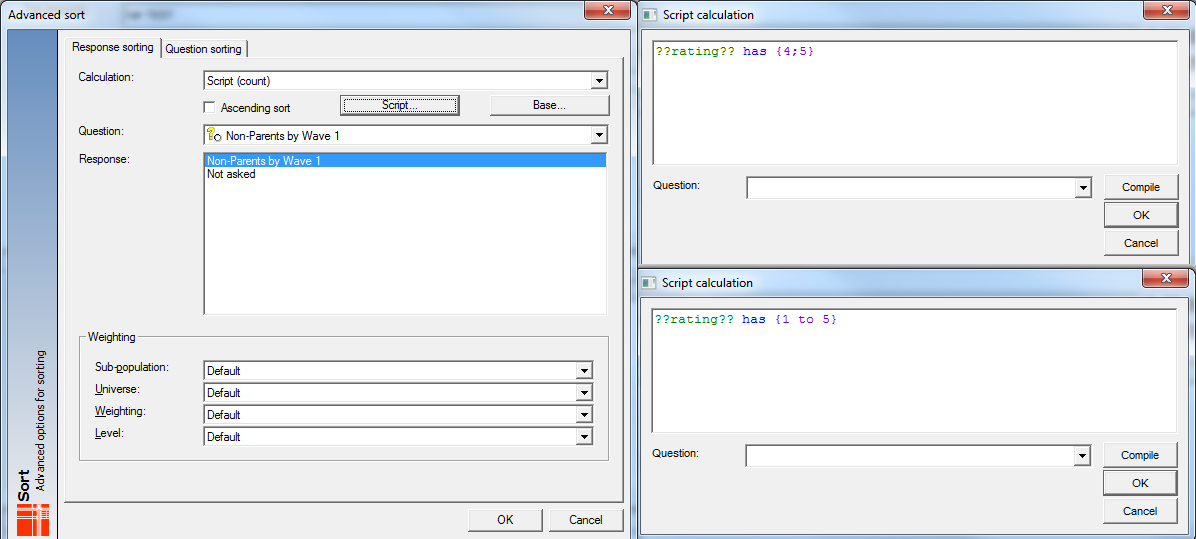Now you can see the below combined table is sorted on Non-Parents Wave 1.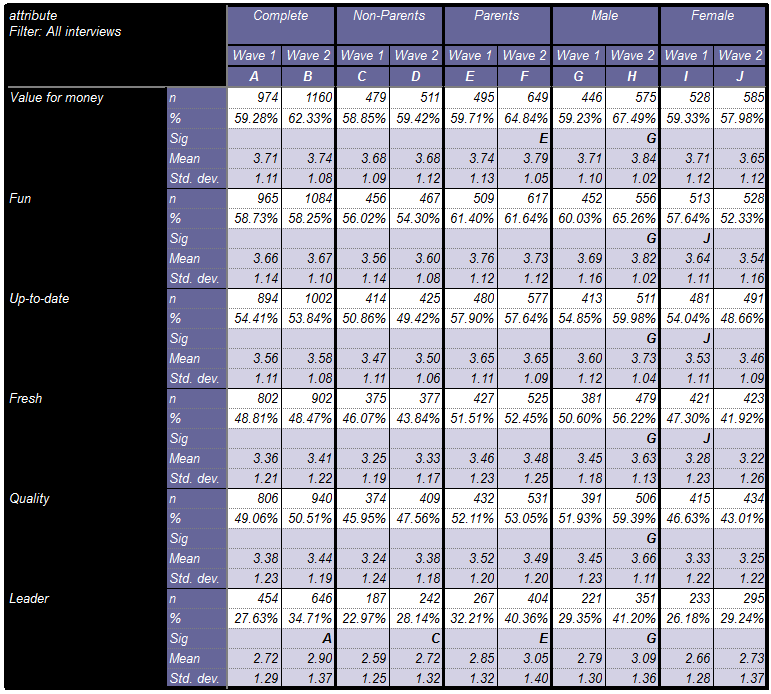Instead of Script (count) you can sort by other calculations e.g. Script (mean), Script (deviation) if required. (See tab definitions in example portfolio: 1.33 & 1.34)

You may be wondering what the ‘Base’ option in the Script (count) calculation does. Well a very brief example is in the Base check tab definition (1.4) in the same example portfolio. (You will need Analyse 5.3.4.0 14/01/2015 onwards to replicate).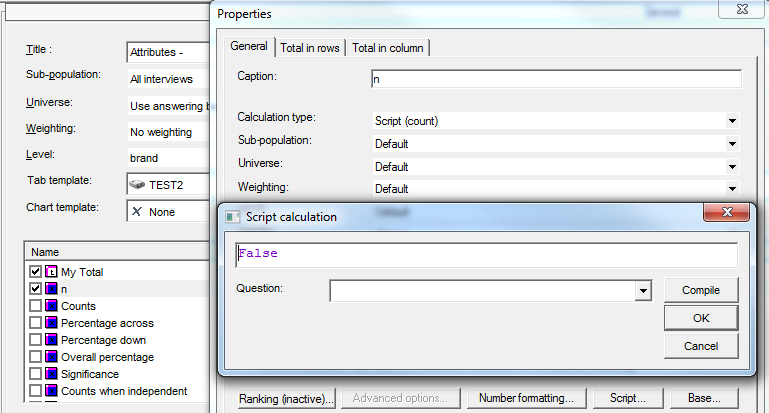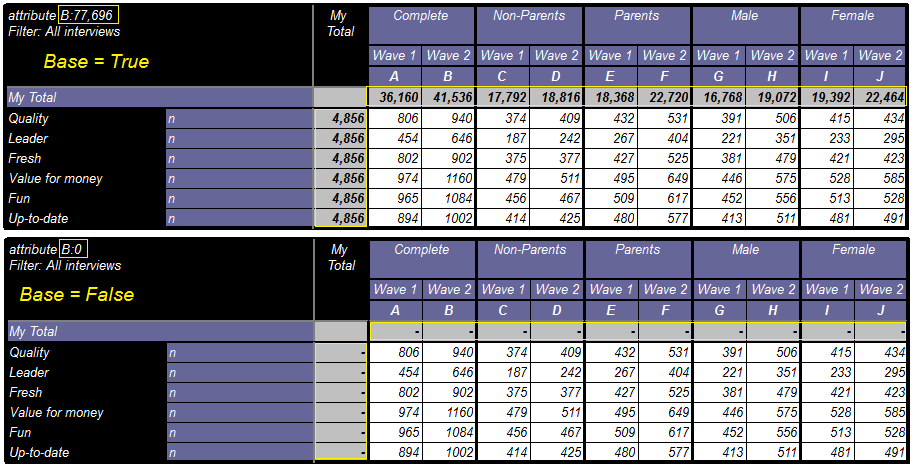0 out of 0 found this helpful
Have more questions? Submit a request

••